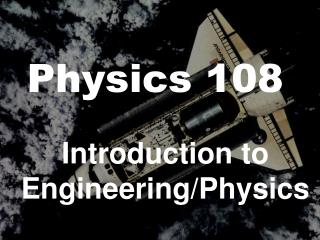DownloadDownload PresentationPhysics 108

# Physics 108

Download Presentation## Physics 108

- - - - - - - - - - - - - - - - - - - - - - - - - - - E N D - - - - - - - - - - - - - - - - - - - - - - - - - - -
##### Presentation Transcript

1. Physics 108 Introduction to Engineering/Physics

2. Objectives of Physics 108 • Learn about the different engineering disciplines • Learn about professionalism in engineering • Become a better problem solver • Develop teaming skills

3. Why Active/Collaborative Learning? • Active • Countless studies have shown improvement in: • short-term retention of material, • long-term retention of material, • ability to apply material to new situations • Collaborative • Engineering teams are used in industry and usually make the best use of time

4. Consulting Astronomy Architecture Mechanical Civil Electrical Why Physics? Engineering

5. Review Units of Measure See Notes

6. Chapter 1 Introduction to Vectors

7. Scalar Quantity • a quantity that has magnitude but not direction • Vector Quantity • a quantity that has both magnitude and direction

8. Vector Quantities • force • e.g. 20 Newtons Eastward • velocity • e.g. 20 meters/second North • acceleration • e.g. 9.8 m/s2 downward

9. Scalar Quantities • length • e.g. 93,000,000 miles • mass • e.g. 180 kg • speed • e.g. 186,000 miles/second

10. Vector • an arrow drawn to scale used to represent a vector quantity • vector notation

11. Resultant • the sum of vectors • Methods of Vector Addition • tip-to-tail method (polygon method) • component method

12. Tip-to-Tail Method • Example 1: Add these vectors using the tip-to-tail method. +

13. + = Tip to Tail Method

14. Notes on Components Components are vectors

15. q Lengths of Components Ax = A cos q Ay = A sin q

16. If Components are Known

17. Unit Vector • magnitude of 1 and a direction